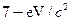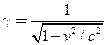Chapter 33, Problem 50PE

Chapter
Section
Textbook Problem

Integrated ConceptsIn supernovas, neutrinos are produced in huge amounts. They were detected from the 1987A supernova in the Magellanic Cloud, which is about 120,000 light years away from the Earth (relatively close to our Milky Way galaxy). If neutrinos have a mass, they cannot travel at the speed of light, but it their mass is small, they can get close. (a) Suppose a neutrino with amass has a kinetic energy of 700 keV. Find the relativistic quantityfor it (b) If the neutrino leaves the 1987A supernova at the same time as a photon and both travel to Earth, how much sooner does the photon arrive? This is not a large time difference, given that it is impossible to know which neutrino left with which photon and the peer ef?ciency of the neutrino detectors. Thus, the fact that neutrinos were observed within hours of the brightening of the supernova only places an upper limit on the neutrino’s mass. (Hint: You may need to use a series expansion to find if for the neutrino, since its (is so large.)

To determine

(a)

The relativistic quantity of 7 eV/c2 neutrinos produced in supernovas.

Explanation

Given:

Kinetic energy of 7 eV/c2 neutrinos is given by 700 keV.

Formula used:

Total energy of any relativistic particle, E=γE0=KE+E0

Where, γ relativistic quantity

E0 rest energy of the particle

KE kinetic energy of the particle

Calculation:

Since total energy, E=γE0=KE+E0

To determine

(b)

The difference in time of travel to earth for photon and neutrino if both are leaving the

supernova at the same time.

Still sussing out bartleby?

Check out a sample textbook solution.

See a sample solution

The Solution to Your Study Problems

Bartleby provides explanations to thousands of textbook problems written by our experts, many with advanced degrees!

Get Started